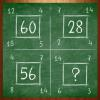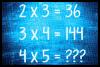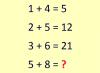BRAIN TEASERSBrain Teasers User Profile

# Aja Romporas

rank
507
points
3
See full ranking list
short ranking list
 505 محمد حيدري 3 506 Ricardo Cardona 3 507 Aja Romporas 3 508 Rosʜʌŋ Zane 3 509 Tóthné Kovács Judit 3
 Replace the question mark with a number MATH PUZZLE: Can you replace the question mark with a number?How much is 4x5? How much is 4x5?Calculate 5+8 If 1+4=5, 2+5=12 and 3+6=21 then 5+8=?